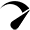# Battery Amp hours To Watts (Ah to Watts) Calculator

Use our battery amp-hour (Ah) to watts calculator to find out the battery capacity in watt-hours.

### How To Use This Calculator?

• Enter the capacity of your battery in amp-hours (Ah).
• Enter the voltage of your battery (12,24,48v).
• Get the result.
Two columns
Vertical
Horizontal

## Watts

{{ field.label }} {{ field.converted }} {{ field.extraView }}
{{ option.label }} {{ option.converted }}
{{ item.label }} {{ item.data.converted }}

Issued on: {{ \$store.getters.getIssuedOn }}
Payment method: {{ \$store.getters.getPaymentType }}
{{ item.label }}: {{ item.converted }}

## How To Calculate Watts In A Battery?

Watts is the unit that represents the total number of power. So to calculate watts from Ah use this formula.

`Watts = Amps*volts`

Multiplying the value of amps with volts will give you the number of watts.

### For Example

Let’s suppose you have a 12v 300Ah battery.

``12v * 300Ah = 3600 watts ``

12v 300Ah battery is equal to 3600 watts or 3.6kW.

## How Many Watts Is A 12-Volt Battery – Chart

Here’s a chart with the conversion of different size 12v batteries in watts.

### Summary

• 12v 7Ah battery is equal to 84 watts.
• 12v 12Ah battery is equal to 144 watts.
• 12v 100Ah battery is equal to 1200 watts or 1.2kW.
• 12v 200Ah battery is equal to 2400 watts or 2.4kW.

## Why Calculate Watts In A 12v Battery?

Battery capacities are rated as Amp-hours (Ah). But most of our appliances are rated as watts.

So calculating Ah to watts will give you an idea of the total capacity of your battery in watts so you can determine how long will your battery last on running any appliances.

Also what appliances running your battery will fall in the safe zone because of battery c-ratings (Charge and discharge rate of battery).

To better understand this, read the article Maximum Charging Current & Voltage For 12v Battery.

Also converting your 12v battery in watts will give you an idea of how many watts it can store so you can pick the right size solar panel in order to recharge your battery.

## How Many Watts Is A 12V Car Battery

Usually, 12v car batteries have a capacity of 60Ah so let’s assume that you have a 12v 60Ah car battery. 12 × 60 = 720 watts. So a 12v car battery is equal to 720 watts.

You can calculate the value by yourself with the formula that I have mentioned above or by using a calculator.

How many watt-hours in a car battery 12v 100Ah car battery has 1200 watt-hours (Wh).

## How Many Watts Are In 12 Volts

To calculate how many watts are 12 volts, you would need the value of amps, and multiplying the amps by 12 will give you

watts (Watts = Amps × 12).

For example, 12v 33Ah how many watts? 12 × 33 = 396 watts

For More Head to roopsolar blogs and get related posts

Scroll to TopOptimized by Seraphinite Accelerator
Turns on site high speed to be attractive for people and search engines.